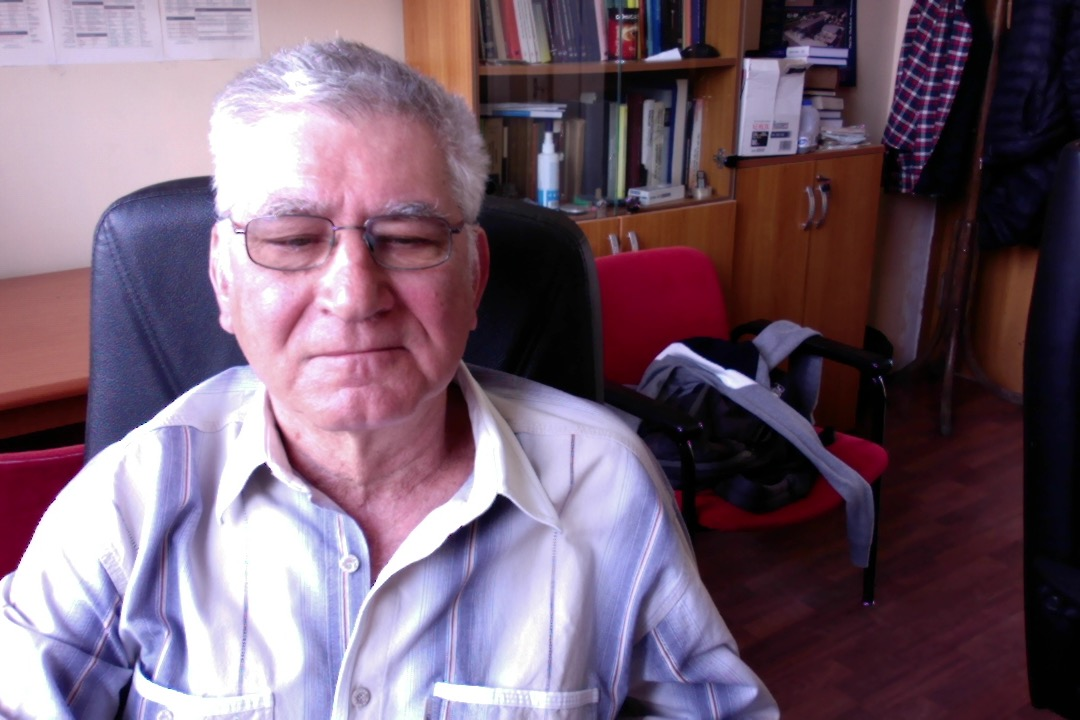##### Dr. Stefan Berceanu
Senior Researcher I (retired)
University of Bucharest, 1976
+40-(0)21-4046247 (ext. 3403)
206
theoretical physics, mathematical physics and mathematics geometrical methods in quantum mechanics
geometrical methods in quantum mechanics, differential geometry, group theoretical methods in physics, coherent states

I have a  program to find a simple geometrical description of coherent states as they are used in Physics:

1) find a geometrical significance for the transition probabilities on manifold of coherent states;
2) find a geometrical description for the phase which appears in the transition amplitude;
3) find all the manifolds for which the angle defined by the scalar product of two normalised coherent states is a distance on the manifold of coherent states;
4) find a geometrical significance for the diastasis function of Calabi in the context of coherent states;
5) state precisely the projective space in which is embedded the manifold of coherent states;
6) state precisely the relationship between the coherent states and geodesics;
7) state precisely the geometrical meaning of the polar divisor (the locus of coherent vectors orthogonal to a fixed coherent vector).
This program has been applied to compact and noncompact hermitian symmetric spaces and now is applied to homogeneous spaces attached to the Jacobi group.